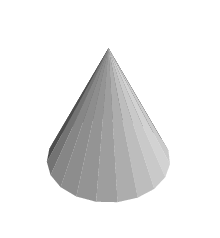# Cone Volume Calculator

This Cone Volume Calculator is designed to help you calculate the volume of a cone. To use the calculator, simply enter the base radius and height of the cone, and click "Calculate". As you enter new dimensions into the volume calculator, a running total will also be displayed.

## Background information and basic definition of a coneA cone is a three-dimensional geometrical shape that narrows from a usually flat, circular base to its highest point, which is known as the apex or vertex.

The following formula can be used to calculate the volume of a cone:

• Cone Volume = (Base x Height) ÷ 3
[Where the base is found by using the formula for the area of a circle, which is pi r2. Here, r represents the radius, which is a straight line that runs from the center of a circle to any point on the circumference.]
• OR
• Cone Volume = 1/3 x π x Radius2 x Height
• Where π is the constant (3.141592654)

Note: Units are provided for convenience purposes only and do not have any impact on the calculations.

Units of the solid objects volume used:

• cubic feet
• cubic meters
• cubic yards

Units of the volume of liquids:

• liters
• quarts
• pints
• gallons